# Velocity Of Sound By Resonance Air Column Tube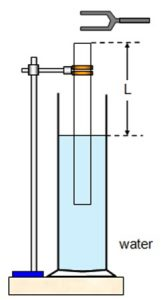The experimental setup of resonance tube experiment to determine the velocity of sound in air is shown in figure, to find the velocity of sound in air the water level in the tube is brought close to the open end of the tube by changing the place of water reservoir. A tuning fork of frequency ‘f’ is set into vibration by striking it against the rubber pad and held it horizontal over the open end. The length of air column in the tube is increased by changing the position of reservoir in doing so, a condition is reached at which the sound heard is maximum, this condition is known as first resonance. If l1 be the first resonating length and l be the wave length of sound when,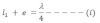If we go on increasing the length of air column in the tube the intensity of the sound heard goes on decreasing first become minimum and then again goes on increasing at a certain length. The intensity of sound becomes maximum again, these condition is called second resonating length. If l2 be the second resonating length,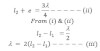If U be the velocity of sound at room temperature.
Then,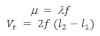Where j is the frequency of sound which is equal to frequency of turning fork,
Velocity of sound at NTP is given by,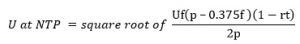where, P-NTP = pressure at NTP
f = aqueous tension
r = cubical expansively of air = 1/273